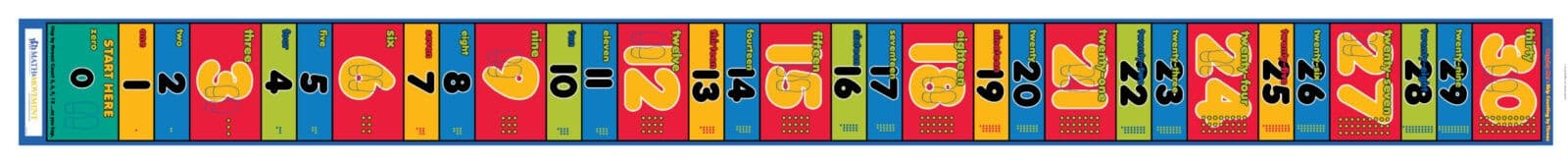## Skip Counting by 3sThe Skip Counting by 3s mat was designed to help students learn the multiples of six and practice multiplying and dividing by three.

Have your students start with the Pre-Test below. Then, spend some time jumping on the mat, using the activities below to guide you!

## Before hopping on the mat:

Ask your child how many of these by problems they know. (Mark it off and try to remember for the final survey!)

## NOW, LET'S TRY SOME ACTIVITIES!

Have a student start on zero. Then, have them point out the pattern that the yellow boxes with the feet follow. Explain to them that these boxes are showing the multiples of six.

Whisper Loud: To begin learning multiples of six, start with the whisper loud technique to have students jump down the mat. Have students follow this pattern: Jump on the one. Whisper, “one.” Jump on the two. Whisper, “two.” Jump on the three. Shout, “THREE.” Jump on the four. Whisper, “four.” Jump on the five. Whisper, “five.” Jump on the six. Shout, “SIX!” Jump on the seven. Whisper, “seven.” Jump on the eight. Whisper, “eight.” Jump on the nine. Shout, “NINE.” Jump on the ten. Whisper, “ten.” Jump on the eleven. Whisper, “eleven.” Jump on the twelve. Shout, “TWELVE!” Continue with this pattern down the mat to thirty.

Skip Counting: Once they are more comfortable with the multiples of six, you can have students skip count down the mat, only jumping on the red boxes. Make sure they are saying the number confidently and correctly when they land on each multiple. If their jumps get ahead of the numbers, have them start again.

Give a student a multiplication by 3s problem (verbally, flashcard, worksheet, etc.).
Example: 3 x 3

The student will begin on 0. They will jump forward 3 times, only landing on the multiples of three. When they land on the sixth number, 9, they will say, “3 x 3 = 9.”

You can also have students practice each multiplication fact as they hop down the mat:
Jump on 3. Say, “3 x 1 = 3.” Jump on 6. Say, “3 x 2 = 6,” etc.

Give a student a division by 3s problem (verbally, flashcard, worksheet, etc.).
Example: 24 ÷ 3

The student will begin on the first number of the problem, 24. Then, they will jump back to zero on the multiples of three. How many jumps did it take to get back to zero? 8! Therefore, 24 divided by 3 is 8.

Give students a dividend that will have a remainder when divided by three. Have them find and stand on the dividend on the mat. Have them jump and count the number of spaces it takes to reach the next lowest multiple of three. The number of spaces is the remainder number. Have them hold it up on their fingers. Then, have them follow the procedure for dividing while keeping the initial “remainder” number on their fingers.

Example: Give them the number 20. They will find and stand on 20 and take two jumps to land on 18. Holding 2 on their fingers, they then jump the multiples to zero, counting how many jumps it takes out loud. When they get to zero, they recite, “20 divided by three is six remainder two.”

Line up two skip counting mats. Tell students you will be comparing these two factors and finding least common multiples. Have one student on each mat. Instruct one student to hop on the multiples going up their mat, shouting out the multiples as they hop. Then, have the second student do the same on the second mat. When the class hears the second student call out a duplicate number, they yell to the second student to stop. Check to see if they found the least common multiple (LCM) or just a common multiple.

Example: Find the LCM of 3 and 6. Line up the Skip Counting by 3s and 6s mats side by side. One student jumps 3, 6, 9, 12, etc. all the way up the mat. Then, the other jumps 6...the class yells, “STOP!”

## After hopping on the mat:

Ask your child how many of these by problems they know now!